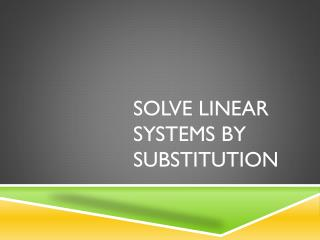DownloadDownload PresentationSolve Linear Systems by Substitution

# Solve Linear Systems by Substitution

Download Presentation## Solve Linear Systems by Substitution

- - - - - - - - - - - - - - - - - - - - - - - - - - - E N D - - - - - - - - - - - - - - - - - - - - - - - - - - -
##### Presentation Transcript

1. Solve Linear Systems by Substitution

2. What does solving a system mean? • In yesterday’s homework, we investigated Cool Copy Company and Bountiful Brochures. • What happened when you graphed the lines? • The lines intersected. • What does that intersection mean? • The two companies sold the same number of copies for the same amount of money. • The point of intersection is called “Solving the System.” • A “System” is a pair of linear equations.

3. 3 ways to solve a system: • Graphing • This method is only successful if you draw an accurate graph. • Substitution • This method has you substitute one equation into another equation and solve. • We will learn this method today. • Elimination • This method is used when the equation is in standard form. • We will learn this method later in the unit.

4. Steps to solve a linear system by substitution • Step 1: Solve one of the equations for one of its variables. • Step 2: Substitute this expression into the other equation and solve for the other variable. • Step 3: Substitute this value into the revised first equation and solve. • Step 4: Check the solution pair in each of the original equations. • Remember, solving a system of linear equations means that you are finding where the two lines intersect.

5. Practice #1: • Find the solution to the linear system: y = x – 34x + y = 32 • Step 1: One of the equations is already solved for one variable, y = x – 3 • Step 2: Substitute “x – 3” in for “y” in the 2nd equation. • 4x + (x – 3) = 32 • 4x + x – 3 = 32 • 5x – 3 = 32 • +3 +3 • 5x = 35 • 5 5 • x = 7 • Step 3: Substitute “7” in for “x” in the first equation, then solve. • y = x – 3 • y = 7 – 3 • y = 4 • The solution to this linear system is an ordered pair, (7, 4). This is where the two lines intersect. • Step 4: Check the solution in each equation. • y = x – 3 • 4 = 7 – 3 • 4 = 4 (true) • 4x + y = 32 • 4(7) + 4 = 32 • 28 + 4 = 32 • 32 = 32 (true)

6. Practice #2: • Find the solution to the linear system: y = x + 43x + y = 16 • Step 1: One of the equations is already solved for one variable, y = x + 4 • Step 2: Substitute “x + 4” in for “y” in the 2nd equation. • 3x + (x + 4) = 16 • 3x + x + 4= 16 • 4x + 4 = 16 • - 4 - 4 • 4x = 12 • 4 4 • x = 3 • Step 3: Substitute “3” in for “x” in the first equation, then solve. • y = x + 4 • y = 3 + 4 • y = 7 • The solution to this linear system is an ordered pair, (3, 7). This is where the two lines intersect. • Step 4: Check the solution in each equation. • y = x + 4 • 7 = 3 + 4 • 7 = 7 (true) • 3x + y = 16 • 3(3) + 7 = 16 • 9 + 7 = 16 • 16 = 16 (true)

7. Practice #3: • Find the solution to the linear system: x – y = 22x + y = 1 • Step 1: Solve the 1st equation for one variable. • x – y = 2 • + y +y • x = 2 + y • Step 2: Substitute “2 + y” in for “x” in the 2nd equation. • 2x + y = 1 • 2(2 + y) + y = 1 • 4 + 2y + y = 1 • 4 + 3y = 1 • -4 -4 • 3y = -3 • 3 3 • y = -1 Use distributive property.

8. Step 3: Substitute “-1” in for “y” in the first equation, then solve. • x – y = 2 • x – –1 = 2 • x + 1 = 2 • -1 -1 • x = 1 • The solution to this linear system is an ordered pair, (1, -1). This is where the two lines intersect. • Step 4: Check the solution in each equation. • x – y = 2 • 1 ––1 = 2 • 1 + 1 = 2 • 2 = 2 (true) • 2x + y = 1 • 2(1) + -1 = 1 • 2 + -1 = 1 • 1 = 1(true)